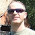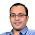Mar 4, 2010

Traffic Jam

You have N cars that are all traveling the same direction on an infinitely long one-lane highway. Unfortunately, they are all going different speeds, and cannot pass each other. Eventually the cars will clump up in one or more traffic jams. In terms of N, what is the expected number of clumps of cars?

Update (05/03/2010):
Solution: Posted by me in comments!! Thanx to Asad (EE, IITB Alumnus) and Siddhant Agarwal (EE, IITB 3rd year student) for their participation in discussion in comments!!

Update (29/01/2011)
Very simple and clear solution posted by wonderwice in comments! Thanks a ton!

1.Assume that when a faster car approaches a slower one it starts moving at the slower speed creating a clump of two cars moving with the speed of slower car.
Thus a clump is created every time a slower car has a faster car following it.
Now assume that the front-most car has speed x. It is a clump in itself. If the car behind it is slower it will give rise to another clump of itself without ever meeting the 1st one else it will join the clump of 1st car.
Now assume that speed of other cars is either >x or <=x with prob. 1/2 each.
Thus expected no. of clumps f[N] = 0.5*[f[N-1]+1]+0.5*f[N-1] =>
f[N] = 1+(N-1)/2

2.@Asad.. I don't think your solution is correct. That's because when the following car is faster, sure it does not add a new clump but the speed of the following car is reduced. You have not included it in your solution.

BTW, The answer I got was (1)+(1/2)+(1/3)+...+(1/N) and I believe its correct.

3.@Asad.. The point I raised in your solution is not really valid. You solution seems correct.

I can apply recursion in the backward direction.

Let E[n] denote expected number of clumps with n cars.

The last car (n-1) cars would be in some clump. The nth car would form another clump if the speed is less than the speed of the clump. Else, one clump extra is formed.

So,
E[n] = 0.5(E[n-1]) + 0.5(E[n-1]+1)
So,
E[n] = E[n-1] + 1/2

But, the answer posted in books I saw is (1)+(1/2)+(1/3)+...+(1/N).

There has to be a mistake in our solution. What is it? :(

4.The problem arises from the assumption that the (n-1)th car has 50% prob of being at a higher speed as compared to the nth car "Irrespective" of the speed of the nth car.

If we assume that the n cars have speeds which come from a finite set e.g (1,2,...,N) and also assume that all cars have different speeds then we get the ans as written in the book i.e 1+1/2+...+1/N (didnt prove it but verified for N=4)

5.Well then it's a matter of assumptions. My approach was to apply the uniformity principle to the speeds if no information is given. Thus if you pick any car, then the speed of any other car will be either less than it or greater with equal prob.
But I implement this problem in excel with speeds ranging uniformly from 1 to 1000 and checking for N=2,3,4,5 and the nos. I get do not match my solution. I guess they are closer to the HP sum solution.

6.@sid. thanx..

@asad.. this is the answer you are getting if you assume uniform distribution. Just thought of an easy example. Three random numbers are chosen uniformly. What is the probability that the graph they form is a pointed one, that is /\ or \/
Ideally, we know its 1-(1/6)-(1/6) = 2/3 {i.e 1-prob(increasing) - prob(decreasing)}.
Let the three numbers be a,b,c. But once two points are chosen (say a and b), according to your method, probability that the three numbers form an increasing sequence is 1/2 {If numbers are a,b,c in order. P(c>b) = 1/2 but P(c>b|b>a) = (1/6)/(1/2) = 1/3}
Hence, the problem. Hope this example clarifies the problem. Thanx Sid.

7.Solution I got from a forum:

Note that the actual speed of car is not important. It is important that which car is travelling faster.

Let X(1), X(2), ...,X(N) be a sequence of random variables over the set {1,2,...,N}, with X(i) different form X(j) if i is different from j. Here X(i) represents the speed of car i, and the set {1,2,...,N} represents the speeds of the cars. We can suppose that the cars, represented by C(1),C(2),...,C(N), are in the order given and move in the East-West (Left To Right) direction. So, if X(N)=1, then there will be exactly one clump, all moving at the speed X(N)=1 of car C(N). If X(N-1)=1, then there will be exactly two clumps, one of which is the right-most car C(N) -- a single-car clump -- having speed greater than 1. The other clump, consisting of (N-1) cars, will be moving behind the car C(N) at the speed X(N-1)=1.

For any k in {1,2,...,N}, the probability P{X(k)=1}=(1/N). If X(k)=1, then all the cars C(1) through C(k) will form a clump and will be moving at the speed X(k)=1. Looking at the cars C(k+1) through C(N), we see that their speeds are all different and any of them can have any of the speeds with equal probability. So, it makes no difference what we assume to be the actual speeds of these cars, what matters is that they be different. So, we may just as well assume the speeds for the cars C(k+1) through C(N) to be {1,2,...,(N-k)}. Also we can rename these cars as A(1),...,A(N-k), so that A(i)=C(k+i) for i in {1,2,...,(N-k)}.

Now let E(N) denote the expected number of clumps of N cars.

It is obvious that E(0)=0.

If X(k)=1, then we get 1 clump consisting of the first k cars (i.e., C(1),...,C(k)). Depending on the value of k, we get on average E(N-k) clumps for the remaining (N-k) cars that are moving ahead of the first k cars.

So,

E(N) = 1 + SUM{(P{X(k)=1})*E(N-k); [k,1,N]}.

Simplifying, we get:

E(N) = 1 + SUM{(1/N)*E(N-k); [k,1,N]},

or

E(N) = 1 + (1/N)*SUM{E(k); [k,1,(N-1)]}.

We can easily show that E(N)=(1/N)+E(N-1), with E(0)=0.

From which we get the Harmonic series E(N)=(1)+(1/2)+(1/3)+...+(1/N).
:)
Finally.. phew!! :)

1.It would be easier if we condition on the position of the fastest car...

8.Here is a simpler way to look at it:

Car number i (ith car from front) is at the head of a clump iff it is slower than all cars that were ahead of it to begin with.

So by linearity of expectation, expected number of clumps, E_N = sum(i=1 to N)P_i

where P_i is the probability that car number i is slower than all cars that were ahead of it to begin with.

P_i is 1/i as the first i cars are all equally likely to be the slowest one.

From here it directly follows that E_N = H_n

9.@wonderwice.. I do not know how I missed your comment! Amazingly simple solution. Thanks a ton :)

10.Like Sid said above, the answer is the sum of the harmonic series ONLY IF the following assumptions are made. They should have been mentioned in the original puzzle question!

1. we assume that the n cars have speeds which come from a finite set e.g (1,2,...,N)

AND

2. we assume that all cars have different speeds

1.Yep. I agree. Thanks

Fraction Brainteaser

Source: Sent to me by Gaurav Sinha Problem: Siddhant writes a Maths test and correctly answers 5 out of 6 Arithmetic questions and 20...